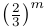# Rolling Advantage with m dice of n sides each

Matt Parker is wrong on the internet and someone must rise to correct him. Alas, today is my day. I shall double reverse-Derek the one and only Matt Parker.

In a recent video, Matt Parker explored a beautiful, geometric way of understanding a problem known to many RPG players. In many RPGs (such as Dungeons and Dragons), players roll a 20-sided die (also called d20, for short) to determine the outcome of an action. In some cases, the dungeon master (who interprets the rules) may decide to give a player “advantage” on a roll, allowing them two roll two d20s (written as 2d20) instead of one and taking the higher of the two. The question that Matt Parker explores is: what is the expected value of taking advantage – how much better is it than just rolling one d20?

He then extends his answer to a more general form:

And this is where he is wrong.

First, consider the case of rolling md1. That is, rolling some number of 1-sided dice. Matt’s formula incorrectly gives:

Obviously since a d1 can only ever return 1 (and so the above should simplify to 1 for all m), something is amiss.

Here I need to define some mathematical notation for clarity. Define a random variable +mdn as the result of taking advantage when rolling m dice, each with n sides.

We can similarly check the case of rolling md2 since this is easy enough to check by hand. The probability of +md2 evaluating to 1 is the probability of only rolling 1s, and so we get:

This is definitely not in line with Matt’s formula. So, what is the actual formula?

The below proof relies on the fact that the calculation for the expectation of a variable can be partitioned into mutually exclusive cases. That is:

Now, suppose that we had some way of representing E(+mdn) and we want to find E(+mdn+1). That is, we want to increase the number of faces of our dice. As we can split our expectation, calculation, we can see:

The first term is the probability that we have no faces of value n+1 times the expected value of taking advantage with mdn. The second term is the probability of having at least one face of value n+1 times that value, as when taking advantage only the largest value is counted.

And now for md3. Trivially, the probability of obtaining zero 3s when rolling m d3s is.

This pattern is suspicious, so let’s try this general form and see if using the iteration pattern preserves it:

Therefore, the expected value of rolling advantage with mdn is:

Through symmetry, the expected value of rolling with disadvantage (taking the lowest die) is: